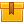# Analog Multimeters

None

## Description

This is the second lesson in the iKNOW® Multimeters Library. This lesson demonstrates various aspects of an analog multimeter, including how to adjust mechanical zero, how to interpret a reading on the voltage and resistance scales, and how to set the function and range switches.

## Objectives

Adjust the mechanical zero of an analog multimeter, Interpret a reading on the voltage scale of an analog multimeter, Interpret a reading on the resistance scale of an analog multimeter, Given an expected measurement, set the function and range switches of an analog multimeter and Adjust the zero on the ohms scale of an analog multimeter.

## ContentThis is the second lesson in the iKNOW™ Multimeters Library. This lesson
demonstrates various aspects of an analog multimeter, including how to
adjust mechanical zero, how to interpret a reading on the voltage and
resistance scales, and how to set the function and range switches.

•Analog Multimeters

0
0 Reviews